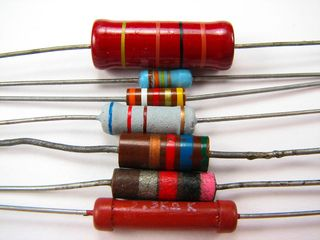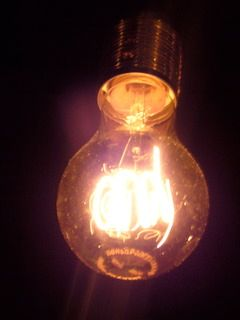Home Practice
For learners and parents For teachers and schools
Textbooks
Full catalogue
Pricing SupportLog in

We think you are located in United States. Is this correct?

# 17.3 Resistance

## 17.3 Resistance (ESAFG)

Resistance is a measure of "how hard" it is to "push" electricity through a circuit element. Resistance can also apply to an entire circuit.

temp text

### What causes resistance? (ESAFH)

On a microscopic level, electrons moving through the conductor collide (or interact) with the particles of which the conductor (metal) is made. When they collide, they transfer kinetic energy. The electrons therefore lose kinetic energy and slow down. This leads to resistance. The transferred energy causes the resistor to heat up. You can feel this directly if you touch a cellphone charger when you are charging a cell phone - the charger gets warm because its circuits have some resistors in them!

Examples of resistorsPhotograph by oskay on Flickr.com

Resistance

Resistance slows down the flow of charge in a circuit. The unit of resistance is the ohm (Ω) which is defined as a volt per ampere of current.

Quantity: Resistance R        Unit: ohm         Unit symbol: ω

$1 \text{ ohm} = 1 \frac{\text{volt}}{\text{ampere}}$

Fluorescent lightbulbs do not use thin wires; they use the fact that certain gases glow when a current flows through them. They are much more efficient (much less resistance) than lightbulbs.

Light bulb filamentPhotograph by clagnut on Flickr.com

All conductors have some resistance. For example, a piece of wire has less resistance than a light bulb, but both have resistance.

A lightbulb is a very thin wire surrounded by a glass housing The high resistance of the small wire (filament) in a lightbulb causes the electrons to transfer a lot of their kinetic energy in the form of heat. The heat energy is enough to cause the filament to glow white-hot which produces light.

The wires connecting the lamp to the cell or battery hardly even get warm while conducting the same amount of current. This is because of their much lower resistance due to their larger cross-section (they are thicker).

An important effect of a resistor is that it converts electrical energy into other forms of heat energy. Light energy is a by-product of the heat that is produced.

There is a special type of conductor, called a superconductor that has no resistance, but the materials that make up all known superconductors only start superconducting at very low temperatures. The “highest” temperature superconductor is mercury barium calcium copper oxide $$(\text{HgBa}_{2}\text{Ca}_{2}\text{Cu}_{3}\text{O}_{\text{x}})$$ which is superconducting for temperatures of $$-\text{140}$$ $$\text{℃}$$ and colder.

#### Physical attributes affecting resistance [NOT IN CAPS]

The physical attributes of a resistor affect its total resistance.

• Length: if a resistor is increased in length its resistance will increase. Typically if you increase the length of a resistor by a certain factor you will increase the resistance by the same factor.

• Width and height or cross-sectional area: if a resistor provides a larger pathway by being made wider or broader then more current can flow through it. If the total surface area through which current flows (cross-sectional area) is increased by a factor the resistance typically decreases by the same factor.

Extension: For a single resistor this can be summarised as

$R \propto \frac{L}{A}$

where $$L$$ is the length and $$A$$ is the cross-sectional area.

#### Why do batteries go flat?

A battery stores chemical potential energy. When it is connected in a circuit, a chemical reaction takes place inside the battery which converts chemical potential energy to electrical energy which powers the electrons to move through the circuit. All the circuit elements (such as the conducting leads, resistors and lightbulbs) have some resistance to the flow of charge and convert the electrical energy to heat and, in the case of the lightbulb, light. Since energy is always conserved, the battery goes flat when all its chemical potential energy has been converted into other forms of energy.

### Resistors in electric circuits (ESAFI)

It is important to understand what effect adding resistors to a circuit has on the total resistance of a circuit and on the current that can flow in the circuit.